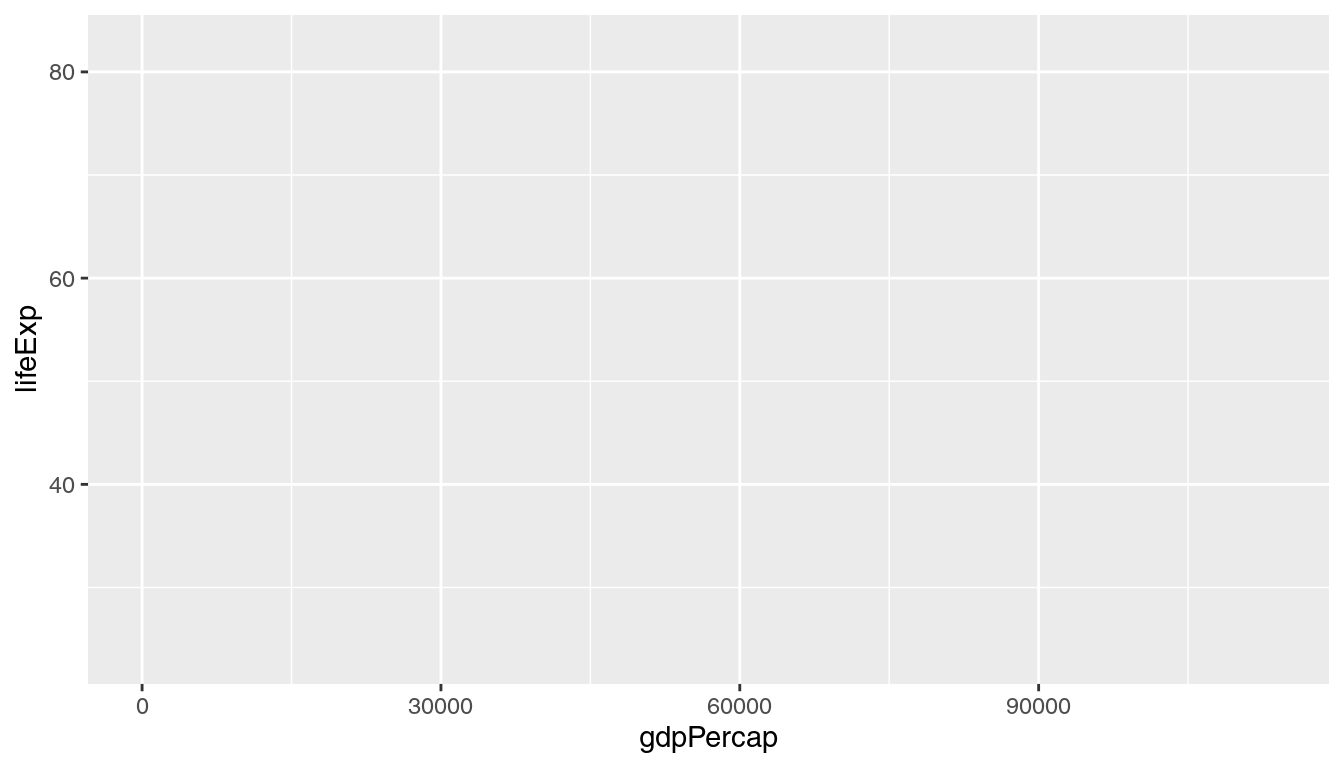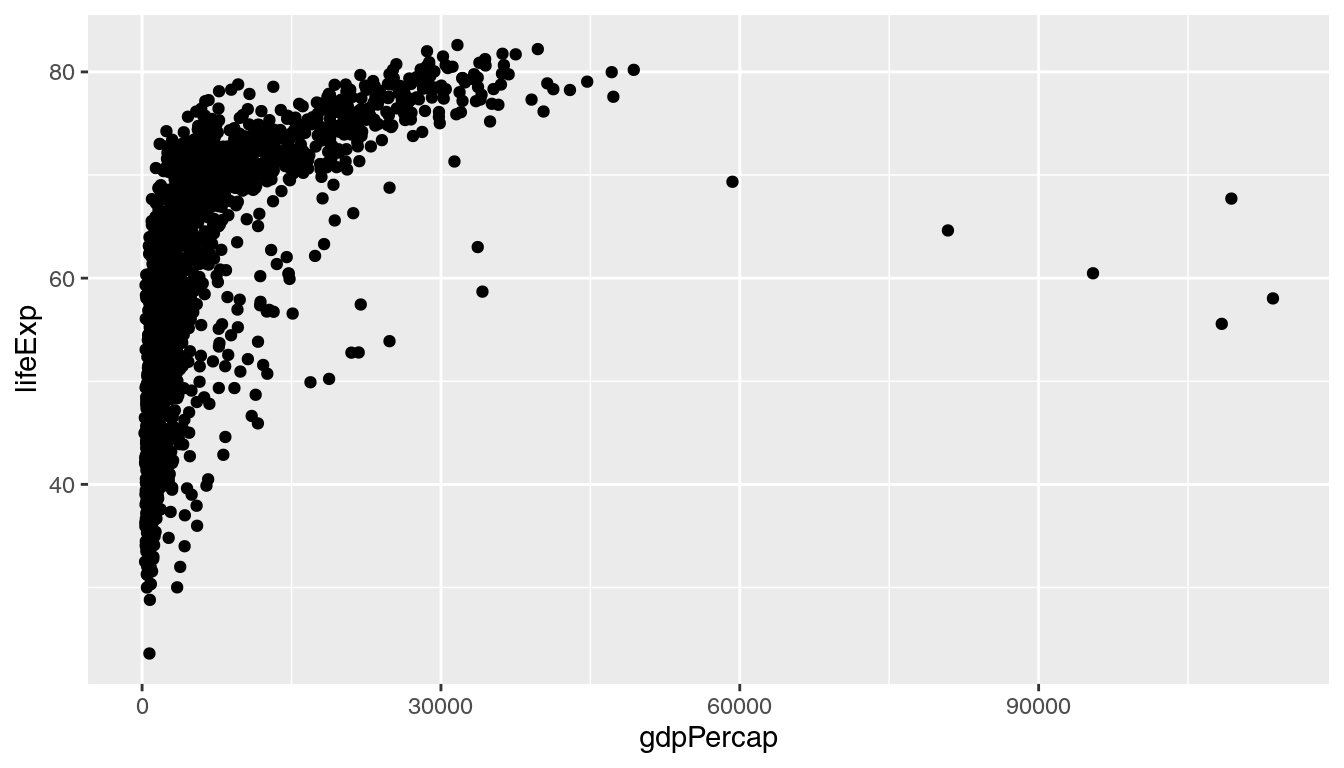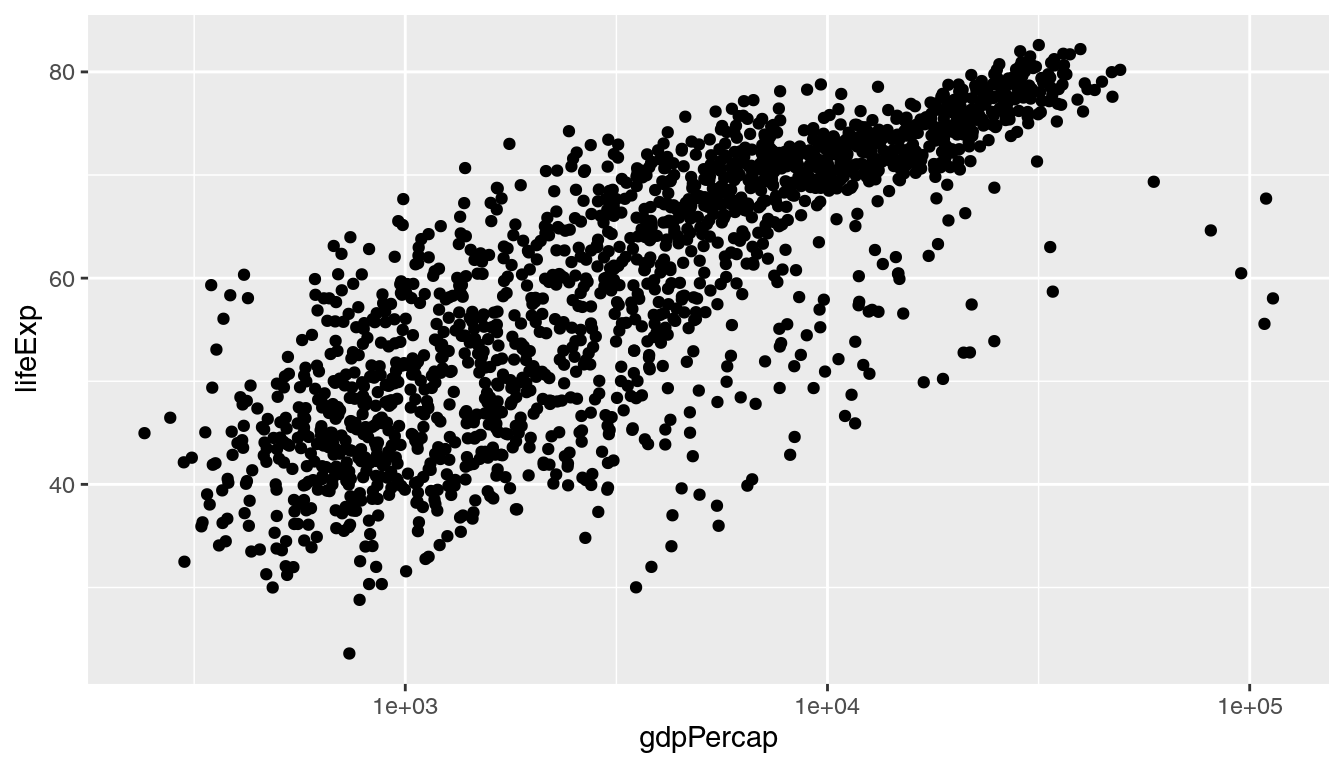An introduction to using the `ggplot` package in R to produce publication-quality graphics.

#### Learning objectives

1. Install and use third-party packages for R
2. Use layering to add elements to a plot
3. Format plots with faceting

## Setup

### Workspace organization

First we need to setup our development environment. We need to create two folders: ‘data’ will store the data we will be analyzing, and ‘output’ will store the results of our analyses.

``````dir.create(path = "data")
dir.create(path = "output")``````

With this workspace organization, we can download the data, either manually or use R to automatically download it. For this lesson we’ll do the latter, saving the file in the `data` directory we just created, and name the file `gapminder.csv`:

``````download.file(url = "http://tinyurl.com/gapminder-five-year-csv",
destfile = "data/gapminder.csv")``````

From this point on, we want to keep track of what we have done, so we will restrict our use of the console and instead use script files. Start a new script with some brief header information at the very top. We want, at the very least, to include:

1. A short description of what the script does (no more than one line of text)
4. The date, preferably in ISO format YYYY-MM-DD
``````# Plot gapminder data
# Jeff Oliver
# jcoliver@email.arizona.edu
# 2017-02-23``````

There are two steps to using additional packages in R:

1. Install the package through `install.packages()`
2. Load the package into active memory with `library()`

For this exercise, we will install the `ggplot2` package:

``````install.packages("ggplot2")
library("ggplot2")``````

It is important to note that for each computer you work on, the `install.packages` command need only be issued once, while the call to `library` will need to be issued for each session of R. Because of this, it is standard convention in scripts to comment out the `install.packages` command once it has been run on the machine, so our script now looks like:

``````# Plot gapminder data
# Jeff Oliver
# jcoliver@email.arizona.edu
# 2017-02-23

#install.packages("ggplot2")
library("ggplot2")``````

## Now plot!

### Scatterplot

For our first plot, we will create an X-Y scatterplot to investigate a potential relationship between a country’s gross domestic product (GDP) and the average life expectancy. We do so by creating a `ggplot` object, then calling `print` on that object:

``````# Load data
stringsAsFactors = TRUE)

# Create plot object
lifeExp.plot <- ggplot(data = gapminder,
mapping = aes(x = gdpPercap, y = lifeExp))

# Draw plot
print(lifeExp.plot)  ``````What happened? There are no points!? Here is where functionality of ggplot is evident. The way it works is by effectively drawing layer upon layer of graphics. So we have established the plot, but we need to add one more bit of information to tell ggplot what to put in that plot area. For a scatter plot, we use `geom_point()`, literally adding this to the ggplot object with a plus sign (+):

``````# Create plot object
lifeExp.plot <- ggplot(data = gapminder,
mapping = aes(x = gdpPercap, y = lifeExp)) +
geom_point()

# Draw plot
print(lifeExp.plot)  ``````It is a little difficult to see how the points are distributed, as most are clustered on the left-hand side of the graph. To spread this distribution out, we can change the scale of the x-axis so GDP is displayed on a log scale by adding `scale_x_log10`:

``````# Create plot object
lifeExp.plot <- ggplot(data = gapminder,
mapping = aes(x = gdpPercap, y = lifeExp)) +
geom_point() +
scale_x_log10()

# Draw plot
print(lifeExp.plot)  ``````One thing of interest is to include additional information in the plot, such as which continent each point comes from. We can color points by another value in our data through the `aes` parameter in the initial call to `ggplot`. So in addition to telling R which data to use for the x and y axes, we indicate which data to use for point colors:

``````# Create plot object
lifeExp.plot <- ggplot(data = gapminder,
mapping = aes(x = gdpPercap, y = lifeExp, color = continent)) +
geom_point() +
scale_x_log10()

# Draw plot
print(lifeExp.plot)  ``````